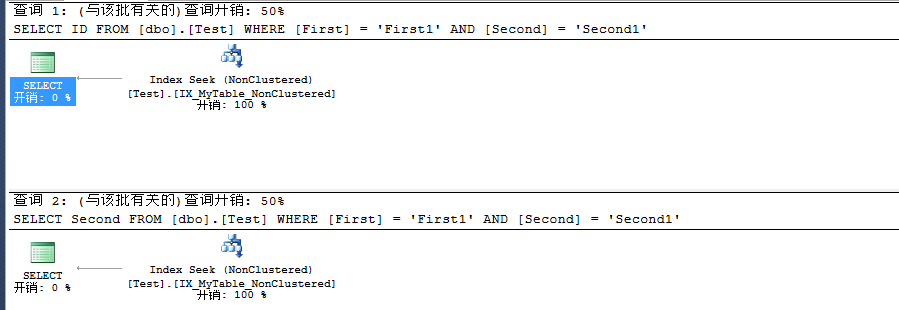# 浅析SQL Server 聚焦索引对非聚集索引的影响

```USE SQLStudy
GO
CREATE TABLE [dbo].[Test](
[ID] [int] NOT NULL,
[First] [nchar](10) NULL,
[Second] [nchar](10) NULL
)
GO```

```INSERT INTO [SQLStudy].[dbo].[Test] ([ID],[First],[Second])
SELECT 1,'First1','Second1'
UNION ALL
SELECT 2,'First2','Second2'
UNION ALL
SELECT 3,'First3','Second3'
UNION ALL
SELECT 4,'First4','Second4'
UNION ALL
SELECT 5,'First5','Second5'
GO```

```CREATE NONCLUSTERED INDEX [IX_MyTable_NonClustered] ON [dbo].[Test] (
[First] ASC,
[Second] ASC
)```

```SELECT ID
FROM [dbo].[Test] WHERE [First] = 'First1' AND [Second] = 'Second1'
SELECT Second
FROM [dbo].[Test] WHERE [First] = 'First1' AND [Second] = 'Second1'
GO
``````CREATE CLUSTERED INDEX [IX_MyTable_Clustered] ON [dbo].[Test] (
[ID] ASC
)```

```SELECT ID
FROM [dbo].[Test] WHERE [First] = 'First1' AND [Second] = 'Second1'
SELECT Second
FROM [dbo].[Test] WHERE [First] = 'First1' AND [Second] = 'Second1'
GO```